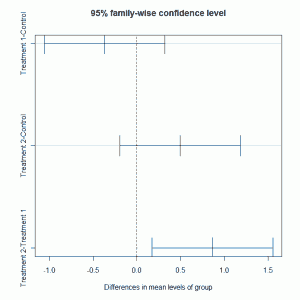[This article was first published on Software for Exploratory Data Analysis and Statistical Modelling, and kindly contributed to R-bloggers]. (You can report issue about the content on this page here)
Want to share your content on R-bloggers? click here if you have a blog, or here if you don't.

In a previous post we considered using R to fit one-way ANOVA models to data. In this post we consider a few additional ways that we can look at the analysis.

In the analysis we made use of the linear model function lm and the analysis could be conducted using the aov function. The code used to fit the model is very similar:

``` > plant.mod2 = aov(weight ~ group, data = plant.df) > summary(plant.mod2) Df Sum Sq Mean Sq F value Pr(>F) group 2 3.7663 1.8832 4.8461 0.01591 * Residuals 27 10.4921 0.3886 --- Signif. codes: 0 ‘***’ 0.001 ‘**’ 0.01 ‘*’ 0.05 ‘.’ 0.1 ‘ ’ 1 ```

The output from using the summary function of the fitted model object shows the analysis of variance table with the p-value showing evidence of differences between the three groups. In R we can investigated the particular groups where there are differences using Tukey’s multiple comparisons:

``` > TukeyHSD(plant.mod2) Tukey multiple comparisons of means 95% family-wise confidence level   Fit: aov(formula = weight ~ group, data = plant.df)   \$group diff lwr upr p adj Treatment 1-Control -0.371 -1.0622161 0.3202161 0.3908711 Treatment 2-Control 0.494 -0.1972161 1.1852161 0.1979960 Treatment 2-Treatment 1 0.865 0.1737839 1.5562161 0.0120064 ```

The multiple comparison tests highlight that the difference is due to comparing treatments 1 and 2. These 95% confidence intervals for the differences shown above can be plotted:

``` plot(TukeyHSD(plant.mod2)) ```

which givesThe post-hoc adjustments are recommended as we are testing after looking at the data rather than undertaking a pre-planned analysis.